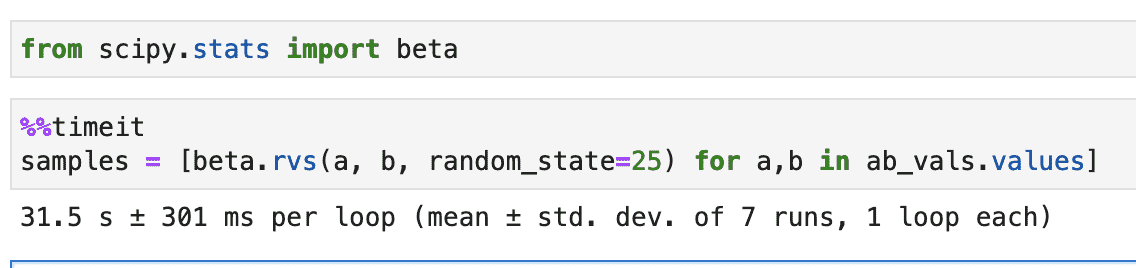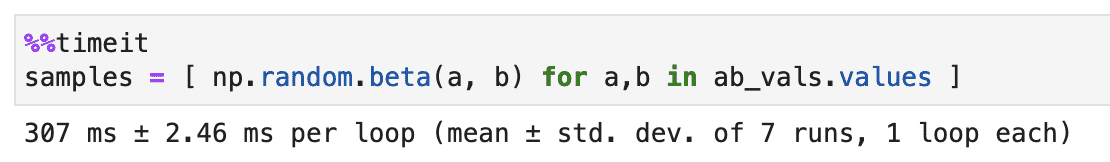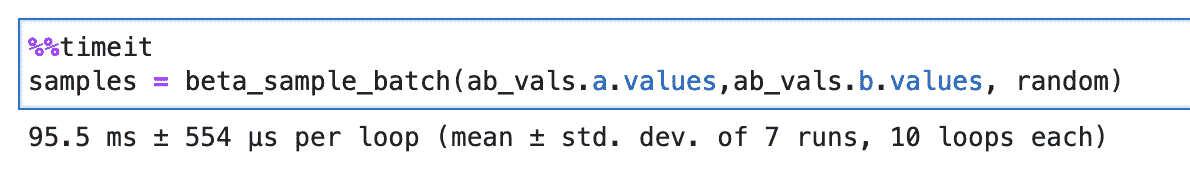Serving recommendation to 200+ millions of users for thousands of candidates with less than 100ms is hard but doing that in Python is harder. Why not add some compiled spice to it to make it faster? Using Cython you can add C++ components to your Python code. Isn’t all machine learning and statistics libraries already written in C and Cython to make them super fast? Yes. But there’s still some optimizations left on the table. I’ll go through how I optimized some of our sampling methods in the recommendation system using C++.

Multi Armed Bandit(MAB) is one of the simpler models in our arsenal, it generally comes down to sampling from Beta distribution or a related distribution using specific distribution parameters for each user and candidate. So recommending for a user is mostly sampling thousands of times from thousands of distributions with different parameters.

Let’s start with a simple sampling code, this is representative of what I started with, before any optimizations.

``````from scipy.stats import beta

samples = [ beta.rvs(a, b, random_state=25) for a,b in ab_vals.values]
``````

Let’s time it for 158k A and B values, sampled from our production data. Realistically we’ll never have to do so many sampling in a single request, but for better comparison in metrics I am using a large number of A and B values.Oh, that’s a lot of time. I hear Numpy is faster than Scipy. Let’s try with Numpy.

``````import numpy as np

samples = [ np.random.beta(a, b) for a,b in ab_vals.values ]
``````That’s 100x faster!. But I had an issue, there was a need to do Percent Point Function(PPF) calculation down the line. Numpy doesn’t support it, so I ended up looking for a better solution. And all these executions are running serially, even though Numpy is built in C and is well optimized we are coming back to Python after each sample. If we could somehow do the sampling in a batch using multiple cores, we can achieve better results.

## Cython and C++#

Cython can help you get away from GIL limitation and run functions in batch, but these functions can only be a pure Cython function or a C/C++ function. I don’t think I am big brain enough to build my own Beta sample and random number generator in Cython. So I went with writing these in C++ with the existing boost library.

`beta_dist_cpp.cpp`

``````#include <boost/math/distributions.hpp>
#include <boost/random.hpp>
#include <iostream>
#include <random>

double beta_sample(double alpha, double beta, long random){
boost::random::mt19937 generator(random);
boost::random::beta_distribution<double> beta_random_dist(alpha, beta);

double random_from_beta = beta_random_dist(generator);
return random_from_beta;
}
//parameters and the random value on (0,1) you drew
int main(){
double a = 1;
double b = 2;
int random = 25;
std::cout<<"Beta for "<<a<<", "<<b<< " Random:"<<random<<" is "<< beta_sample(a,b,random);
}
``````

So from our old Scipy code, we were calling `rvs` with a random state. From its docs, we can see its creating Numpy’s RandomState from that number. Which is a container for Mersenne Twister. This is what our first line in our C++ function is doing, setting up a mersenne twister (pseudo) random number generator. The next line is creating beta distribution using A and B values. Boost has two implementations of Beta distribution. One in `boost::random` another in `boost::math`. The first is used for random sampling, that is what we want to do, the second for other statistics like PPF, Kurtosis etc. We need the second one too for calculating PPF but I’m not including that in this example (refer docs to see how to do it). The 3rd line is actually doing the sampling, I referred to this stackoverflow answer to understand how to sample in boost.

Building the C++ code, make sure you have boost installed.

``````\$ g++ -o beta_dist.out beta_dist_cpp.cpp -I/usr/local/include/boost
\$ ./beta_dist.out
Beta for 1, 2 Random:25 is 0.371978
``````

That’s it. We have pure C++ function that we can parallelize in Cython. Let’s write a Cython wrapper and build into Python importable function

`beta_dist.hpp`

``````#ifndef BETA_DIST_H
#define BETA_DIST_H

#include <boost/math/distributions.hpp>
#include <boost/random.hpp>
#include <iostream>
#include <random>

double beta_sample(double, double , long);
#endif
``````

`beta_dist.pyx`

``````#!python
#cython: language_level=3
import numpy as np
from cython.parallel import prange
cimport cython

cdef extern from "beta_dist.hpp" nogil:
double beta_sample(double, double , long)

@cython.boundscheck(False)
@cython.wraparound(False)
def beta_sample_batch(double[:] a, double[:] b, long[:] random):
cdef int N = len(a)
cdef double[:] Y = np.zeros(N)
cdef int i
for i in prange(N, nogil=True):
Y[i] = beta_sample(a[i], b[i], random[i])
return Y
``````

In the wrapper `pyx` we are importing the beta_sample from C++ and writing a pure Cython function that takes 3 vectors and runs beta_sample for them in parallel using `prange`. `prange` also takes number of threads as input, if not passed it takes it from OMP_NUM_THREADS or defaults to number of CPU cores.

You’ll need a `setup.py` to build the C++ and Cython code to make it importable in Python

`setup.py`

``````from distutils.core import setup
from Cython.Build import cythonize
from distutils.extension import Extension
from Cython.Distutils import build_ext

import pathlib
module_path = pathlib.Path(__file__).parent.resolve()
ext_modules = [
Extension("beta_dist",
[str(module_path/"beta_dist.pyx"), str(module_path/"beta_dist_cpp.cpp")],
libraries=["m"],
include_dirs=["/usr/local/include/boost"],
library_dirs=["/usr/local/lib"],
extra_compile_args = ["-O3", "-ffast-math", "-march=native", "-fopenmp" ],
language="c++")
]
temp_build = module_path/"build"
setup(
name="beta_dist",
cmdclass = {'build_ext': build_ext},
ext_modules=ext_modules,
script_name = 'setup.py',
script_args = ['build_ext', f'--build-lib={module_path}', f'--build-temp={temp_build}'],
)
``````

Build the Cython extensions. Make sure you have Cython. You might also have to install OpenMP if you are not using GCC.

``````python setup.py build_ext --inplace
``````

Let’s see what’s the improvement is

``````from beta_dist import beta_sample_batch

random = np.random.randint(low=0, high=1000, size=158208)
samples = beta_sample_batch(ab_vals.a.values,ab_vals.b.values, random)
``````That’s 3x faster than even our Numpy run on a 4 core machine while retaining the ability to add PPF calculation (I did add it and results were pretty much the same). Since its using all cores, it’ll be even better on larger machines.

So overall we were able to achieve 340x speedup from Scipy code while retaining all the capability of the Scipy code.

That’s it? Why not go ham and implement pre and post processing in Cython as well? Shiv did it. That’s not ham enough, why not implement the whole model in a compiled language? We did that too. Golang, Rust.